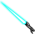Thread: Stat Equations View Single PostFoyle
 07.26.2019 , 11:08 AM | #2 Quote I assumed that what dipstik calls coefficients a and b wouldn't change, since it serves as a limes, cap which we can't exceed, and changing it without adjusting all NPC attacks everywhere would dramatically change balance in old content. Assuming only the last coefficient in exponent changed, I got the new defense coefficient 2.125 instead of 1.2: def_chance = 30*(1-(1-0.01/0.3)^((def_rating/75)/2.125)) This matches tooltip data (CSV) for 10 data points in range between 560 to 2800. Shield was more difficult, because working under the same assumption I can't fit the curve to all data points, one doesn't match- this might be a result of rounding error, but I can't be sure. New coefficient would be 1.2789 instead of 0.78: shld_chance = 50*(1-(1-0.01/0.5)^((shld_rating/75)/1.2789)) This matches tooltip data (CSV) in range between 431 to 4243 rating, so even if the formula is wrong, it is reasonably accurate in the entire interesting range. Interestingly, shield curve normalized to stat budget is almost identical to L70 formula and gear, and defense curve is flatter by up to one ehancement's worth: https://www.dropbox.com/s/866l47wa8g...stion.png?dl=0 https://www.dropbox.com/s/7yunujplss...stion.png?dl=0